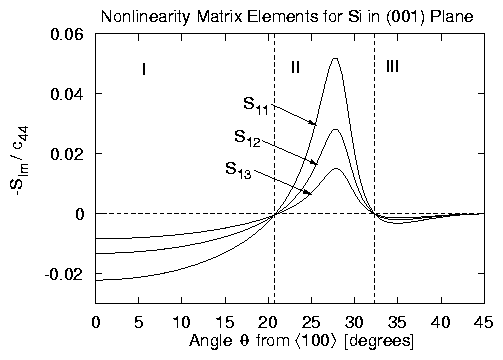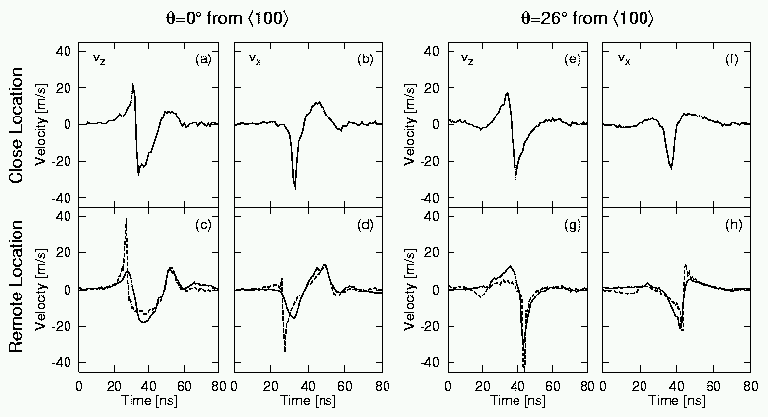Dependence of surface acoustic wave nonlinearity on propagation direction in crystalline silicon

# Dependence of surface acoustic wave nonlinearity on propagation direction in crystalline silicon

## Abstract

Nonlinear distortion of a surface acoustic wave in a crystal depends not only on the second- and third-order elastic constants, but also on the plane and direction of propagation. A theory developed recently for nonlinear surface waves in arbitrary anisotropic solids reveals a strong dependence on propagation direction in the (001) plane of crystalline silicon. Specifically, the longitudinal velocity waveform develops compression shocks in some directions, rarefaction shocks in others. Measurements of laser-generated pulses support these predictions.

Note: A Postscript version of each figure in the text may be downloaded by selecting the figure.

## Theory and Experiment

The anisotropy of the elastic properties of crystalline materials causes the propagation of surface acoustic waves (SAWs) to vary as a function of direction. The theory employed here was developed by Hamilton et al.  for arbitrary crystalline symmetries and follows the approach used by Zabolotskaya  for nonlinear Rayleigh waves in isotropic media. The resulting model equations for the jth vector component (j = x,y,z) of particle velocity are given by
 vj(x,z,t) = ¥ å n = -¥ vn(x)unj(z)ein(kx-wt) , dvn dx +an vn = n2w 2rc4 å l+m = n lm |lm| Slmvlvm ,
where x is the direction of propagation, z is the coordinate normal to the traction-free surface of the solid, w is the fundamental angular frequency, k is the corresponding wavenumber in the expansion, r is the density, c is the SAW speed, unj(z) are eigenfunctions of the linear problem, and Slm is a nonlinearity matrix that is known explicitly in terms of the second- and third-order elastic constants of the material. The coupled equations for vn(x) are solved numerically, with the ad hoc absorption coefficients an = n2a1 introduced for numerical stability when shocks are formed. One of the advantages of the theory is that it has no adjustable parameters, and no curve fitting need be employed. For all calculations below, the elastic constants for silicon were taken from the measurements reported by McSkimin and Andreatch . However, neither the theoretical nor experimental techniques described below are limited to this material.Figure 1: Nonlinearity matrix elements S11, S12, S13 for crystalline silicon in the (001) plane as a function of direction. Because of the symmetries of this cut, the matrix elements are symmetric about q = 45° and periodic every 90°. The matrix elements Slm are normalized by the second-order elastic constant c44.Figure 2: Comparison of experiment (solid lines) and theory (dashed lines) for surface acoustic wave pulses propagating in the (001) plane of crystalline silicon in the directions q = 0° (Region I) and q = 26° (Region II) from á100ñ, from the location close to the excitation region (upper row) to the remote location 14.6 mm away (lower row). Here, vz and vx are the vertical (left columns) and longitudinal (right columns) velocity waveforms.

The nonlinearity matrix elements indicate the coupling strength between pairs of harmonics, e.g., Slm describes how energy is transferred from the lth and mth harmonics to the (l+m)th harmonic. The elements S11, S12, S13 are plotted in Figure 1. The nonlinearity divides into three distinct regions in terms of the angle q between the direction of propagation and á100ñ direction. In Region I (0° £ q < 21°), the nonlinearity is ``negative.'' This means that positive segments of the longitudinal particle velocity waveform steepen backward in space, and negative segments steepen forward (i.e., opposite what a sound wave does in air or water). In Region II (21° < q < 32°), the nonlinearity is ``positive,'' with waveform distortion the reverse of that in Region I. In Region III (32° < q £ 45°), the nonlinearity is again ``negative,'' although the surface wave behaves increasingly like a shear wave for q®45°, and the nonlinearity is very weak. Finally, Figure 1 indicates that S11, S12, S13 go to zero at q @ 21° and q @ 32°. Additional calculations show that all elements are close to zero at these angles, and hence propagation is expected to be nearly linear in both these directions even for finite amplitude waves.

To generate SAWs with a sufficiently large amplitude to exhibit nonlinear effects, a pulsed laser technique was employed. Using this technique, several authors have reported data with significant harmonic generation and shock formation in both isotropic [4,5,6] and crystalline [6,7] media. Additional measurements are reported here for two directions in the (001) plane of crystalline silicon. Excitation was accomplished using a Nd:YAG laser of wavelength 1064 nm, pulse duration 7 ns, and energy up to 50 mJ that was focused into a thin strip 6 mm by 50 mm. A strongly absorbing carbon layer in the form of an aqueous suspension was placed on the surface in the excitation region to intensify SAW excitation. The transient SAW waveforms were measured absolutely with a calibrated probe beam deflection setup using stabilized cw Nd:YAG laser probes of wavelength 532 nm and power 40 mW that were focused into spots 4 mm diameter and located 14.6 mm apart. The reflected signals were detected by two photodiodes, the output of which is proportional to the vertical velocity component vz at the surface. The detection bandwidth was limited to 500 MHz. The resulting SAW pulses were typically 30-40 ns duration with peak strains up to 0.005. The effects of diffraction were determined to be insignificant.

## Results

Figure 2 shows SAW pulses in the directions q = 0° [(a)-(d)] and q = 26° [(e)-(h)] with respect to the á100ñ direction. The top row of plots shows measured waveforms at the probe location close to the source, while the bottom row shows measured (solid) and predicted (dashed) waveforms at the remote location 14.6 mm away. The left column of each set gives the directly measured vertical velocity vz, while the right column gives the longitudinal velocity vx computed from linear theory. In both cases, the absorption coefficient at the peak frequency was selected to be (100[`(x)])-1, where [`(x)] is the estimated shock formation distance for that case.

A comparison of experiment and theory verify that at least two distinct regions of nonlinearity exist in this surface cut. First, consider the q = 0° case. In Region I, the coefficient of nonlinearity is negative. Hence in the vx waveform the peaks travel slower than the SAW speed, and troughs travel faster. This behavior can be most easily seen in (b) and (d), where the trough becomes shallower as it advances and the pulse evolves into an inverted N wave. It is also seen in the pulse lengthening of the waveforms as the trough and peak move apart in time. In contrast, consider the q = 26° case. In Region II, the coefficient of nonlinearity is positive. Hence in the vx waveform the peaks travel faster than the SAW speed, and troughs travel slower. This behavior can be most easily seen in (f) and (h), where the nonlinearity causes the waveforms to develop into a sawtooth wave. This evolution is similar to that in a fluid, except for the cusping near the shock front, which is a well-known characteristic of nonlinear SAWs .

In conclusion, these results demonstrate that (1) the nonlinearity of SAWs in the (001) plane of crystalline silicon is strongly dependent upon the direction of propagation, and (2) at least two distinct regions of nonlinearity exist in this surface cut. These are also the first reported measurements of such angular dependence.

## Acknowledgments

Yu. A. Il'inskii and E. A. Zabolotskaya are thanked for helpful discussions of this work. The experiments were performed while one of the authors (AL) was on leave at the University of Heidelberg with financial support from Volkswagen-Stiftung, Deutche Forschungsgemeinschaft, and the Russian Foundation for Basic Research. The theoretical work was supported by the U. S. Office of Naval Research.

## References


Hamilton, M. F., Il'inskii, Yu. A., and Zabolotskaya, E. A., J. Acoust. Soc. Am. 105, 639 (1999).

Zabolotskaya, E. A., J. Acoust. Soc. Am. 91, 2569 (1992).

McSkimin, H. J. and Andreatch, Jr., P., J. Appl. Phys. 35, 3312 (1964).

Lomonosov, A., et al., J. Acoust. Soc. Am. 105, 2093 (1999).

Kolomenskii, Al. A., et al., Phys. Rev. Lett. 79, 1325 (1997).

Lomonosov, A. and Hess, P., in Proc. 14th ISNA, ed. by Wei, R. J. (Nanjing University Press, Nanjing, 1996), pp. 106-111.

Kumon, R. E. et al., in Proc. 16th ICA & 135th ASA Meeting, ed. by Kuhl, P. K. and Crum, L. A. (ASA, Woodbury, New York, 1998), Vol. 3, pp. 1557-1558.

Ronald Kumon / UT-Austin / Created 09 Jul 1999 / Updated 09 Jul 1999

File translated from TEX by TTH, version 2.00. This page is best viewed by browsers that support HTML 3.2 or higher (Netscape 3.0+, IE 3.0+). If you have problems viewing the text or symbols (e.g., the Greek letter xi, x, should appear here), please check your document encoding; see the TTH web site for suggested solutions for your platform.